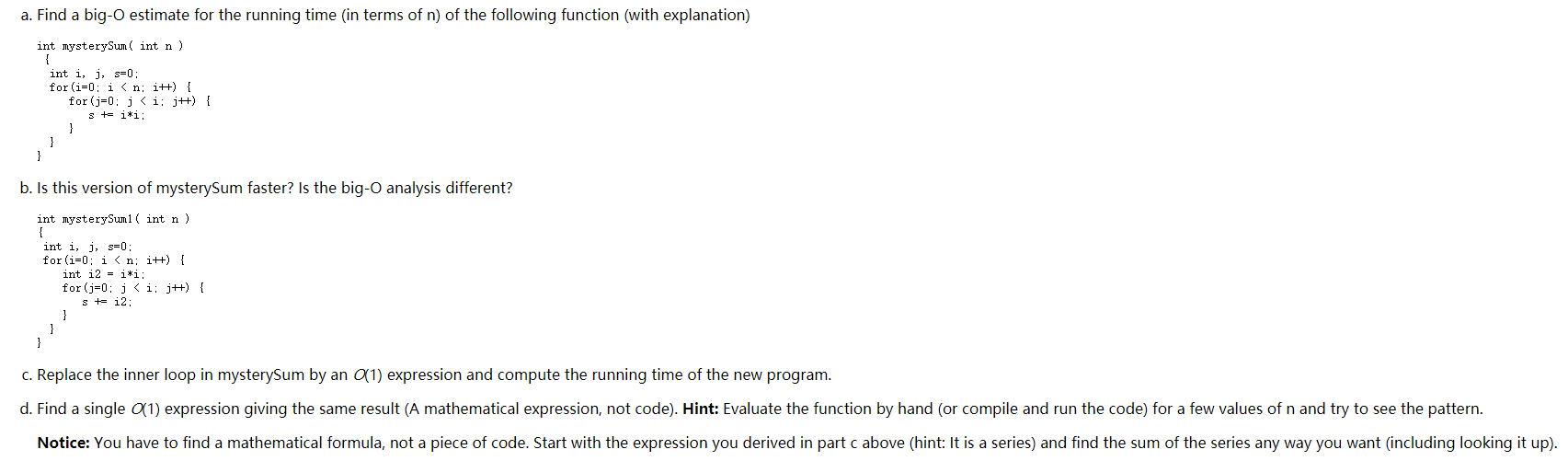Home / Answered Questions / Other / a-find-a-big-o-estimate-for-the-running-time-in-terms-of-n-of-the-following-function-with-explanatio-aw349

# (Solved): A. Find A Big-O Estimate For The Running Time In Terms Of N) Of The Following Function (with Explana...

Q: Question about Big-O Analysis.a. Find a big-O estimate for the running time in terms of n) of the following function (with explanation) int mysterySum( int n) int i, j, s=0; for(i=0; i<n; i++) { for(j=0; j<i; j++) { s += i*i; b. Is this version of mysterySum faster? Is the big-O analysis different? int mysterySumi( int n) int i, j, s=0; for(i=0; i<n; i++) { int i2 = i*i; for(j=0; j <i; j++) { s += i2: c. Replace the inner loop in mysterySum by an 91) expression and compute the running time of the new program. d. Find a single 91) expression giving the same result (A mathematical expression, not code). Hint: Evaluate the function by hand (or compile and run the code) for a few values of n and try to see the pattern. Notice: You have to find a mathematical formula, not a piece of code. Start with the expression you derived in part c above (hint: It is a series and find the sum of the series any way you want (including looking it up).

We have an Answer from Expert